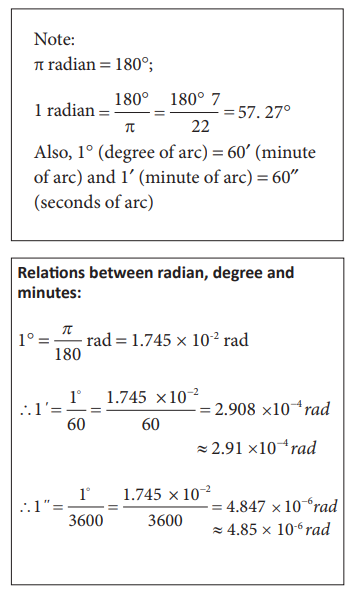Home | | Physics 11th std | Measurement

# Measurement

The comparison of any physical quantity with its standard unit is known as measurement.

MEASUREMENT

The comparison of any physical quantity with its standard unit is known as measurement.

Measurement is the basis of all scientific studies and experimentation. It plays an important role in our daily life. Physics is a quantitative science and physicists always deal with numbers which are the measurement of physical quantities.

“When you can measure what you are speaking about and can express it in numbers, you know something about it; but when you cannot express it in numbers, your knowledge is of a meager and unsatisfactory kind” - Lord Kelvin

## Definition of PhysicalQuantity

Quantities that can be measured, and in terms of which, laws of physics are described are called physical quantities. Examples are length, mass, time, force, energy, etc.

## Types of Physical Quantities

Physical quantities are classified into two types. There are fundamental and derived quantities.

Fundamental or base quantities are quantities which cannot be expressed in terms of any other physical quantities. These are length, mass, time, electric current, temperature, luminous intensity and amount of substance.

Quantities that can be expressed in terms of fundamental quantities are called derived quantities. For example, area, volume, velocity, acceleration, force.

## Definition of Unit andits Types

The process of measurement is basically a process of comparison. To measure a quantity, we always compare it with some reference standard. For example, when we state that a rope is 10 meter long, it is to say that it is 10 times as long as an object whose length is defined as 1 metre. Such a standard is known as the unit of the quantity. Here 1 metre is the unit of the quantity ‘length’.

An arbitrarily chosen standard of measurement of a quantity, which is accepted internationally is called unit of the quantity.

The units in which the fundamental quantities are measured are called fundamental or base units and the units of measurement of all other physical quantities, which can be obtained by a suitable multiplication or division of powers of fundamental units, are called derived units.

## Different types ofMeasurement Systems

A complete set of units which is used to measure all kinds of fundamental and derived quantities is called a system of units. Here are the common system of units used in mechanics:

a. the f.p.s. system is the British Engineering system of units, which uses foot, pound and second as the three basic units for measuring length, mass and time respectively.

b. The c.g.s system is the Gaussian system, which uses centimeter, gram and second as the three basic units for measuring length, mass and time respectively.

c. The m.k.s system is based on metre, kilogram and second as the three basic units for measuring length, mass and time respectively.

## SI unit System

The system of units used by scientists and engineers around the world is commonly called the metric system but, since 1960, it has been known officially as the International System, or SI (the abbreviation for its French name, Systeme International). The SI with a standard scheme of symbols, units and abbreviations, were developed and recommended by the General Conference on Weights and Measures in 1971 for international usage in scientific, technical, industrial and commercial work. The advantages of the SI system are,

i.               This system makes use of only one unit for one physical quantity, which means a rational system of units

ii.               In this system, all the derived units can be easily obtained from basic and supplementary units, which means it is a coherent system of units.

iii.               It is a metric system which means that multiples and submultiples can be expressed as powers of 10.

In SI, there are seven fundamental units as given in Table 1.2Triple point of water is the temperature at which saturated vapour, pure and melting ice are all in equilibrium. The triple point temperature of water is 273.16K

Table 1.3 lists some of the derived quantities and their units.Tags : Definition and Types of Physical Quantity, Definition and Types of Unit, Different types of Systems, SI unit System , 11th Physics : UNIT 1 : Nature of Physical World and Measurement
Study Material, Lecturing Notes, Assignment, Reference, Wiki description explanation, brief detail
11th Physics : UNIT 1 : Nature of Physical World and Measurement : Measurement | Definition and Types of Physical Quantity, Definition and Types of Unit, Different types of Systems, SI unit System